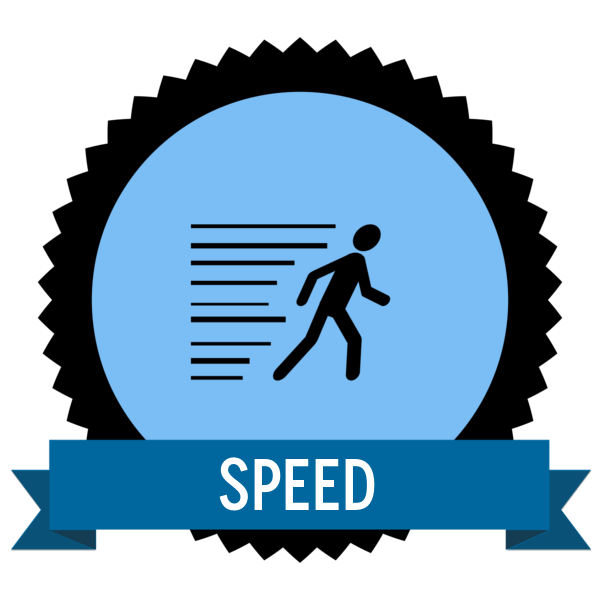# Speed

0 Points

The badge is all about a handy scientific equation; the one that relates speed distance and time. Since speed equals distance over time, one can easily work out speed given how far an object has travelled and how long it took and many of you will be able to rearrange this equation to work out either distance travelled or time taken if you’re given values for the other two. Acceleration is the next logical step once you have studied speed and those car fans among you ought to know lots about that!

To achieve this badge you must be able to:

• Define speed, acceleration, average speed and relative motion
• Quote the formula: speed equals distance over time verbally and write it down as an equation and a formula triangle
• Use the formula or triangle to calculate any of the unknowns unaided
• Label a distance time graph for a journey to show what constant speed and acceleration looks like

Here’s the type of graph you should be able to label:

Follow